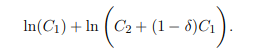# HE3002 MACROECONOMICS Assignment, NTU, Singapore: Consider A Two-Period, Small Open Economy With Durable Consumption Goods

HE3002 Take-home Quiz

1. Consider a two-period, small open economy with durable consumption goods. The purchase of durable consumption goods in period 1, denoted C1, continues to provide utility in period 2. The utility flow households receive from the stock of durables in period 2 depends on new purchases of durables in period 2, C2, and on the undepreciated stock of durables pruchased in period 1. Durable consumption goods are assumed to depreciate at rate δ ∈ (0, 1). Preferences are described by the following utility functionAssume that the initial asset position B0 is zero. Q1 and Q2 denote the endowment in period 1 and 2, respectively. Suppose that in period 1 the country experiences a persistent increase in output. Specifically, assume that endowment increases by 1 in period 1 and by ρ ∈ (0, 1) in period 2. Find the conditions on (δ, ρ) such that the changes in trade balance in period 1 is negative, and changes in C1 and C2 are positive.

2. Consider a two-period small open economy populated by a large number of
identical households with preferences described by the utility function
ln(C1) + ln(C2 − αC1),

where α ∈ (0, 1) is a parameter measuring the degree of habit formation. Households are endowed with Q > 0 units of consumption goods each period and can borrow or lend at the world interest rate r. Households start period 1 with no assets or debts from the past (B0 = 0). Calculate the equilibrium levels of consumption and trade balance in period 1 as functions of (α, Q, r∗).

3. Consider a three-period, small open production economy, where household’s lifetime utility function is given as:
ln(C1) + β ln(C2) + β 2 ln(C3).

The production function in each period is given as Qt = AtI 1/2 t−1
, where we assume capital fully depreciates each period. Every household enters period 1 with a zero asset position (Bh 0 = 0). Suppose that the world interest rate is constant over time at r ∗ = 0.1. Let A1 = 1, A2 = 2, A3 = 3, I0 = 1 4
, r0 = 0, and β = 0.9. Compute the equilibrium levels of consumption, trade balance, and current account in periods 1,2 and 3.

4. Consider a two-period, small open economy populated by a large number of households with preferences captured by the following lifetime utility function